# Advanced Encryption Standard (AES) in Cryptography

Cryptography | Advanced Encryption Standard (AES): In this tutorial, we will learn about the advanced encryption standard (AES), its steps of encryption and decryption, its features, working, and types of messages in the AES. By Monika Sharma Last updated : May 25, 2023

The original name of Advanced Encryption Standard (AES) is Rijndael is a specialist for the encryption of electronic data which is established by the U.S.(NIST) as the National Institution of Standard and Technology in 2001.

It is based on a design principle known as a substitution permutation network in cryptography and is efficient in both software and hardware. Unlike, its precursor
DES, AES does not use a Feistel structure as DES. AES is a version of Rijndael which has a fixed block size of the message as 128 bits, and the key size is 128, 192, or 256 bits. As like, Rijndael per se is particular with block and key sizes that may be multiple of 32 bits, with a minimum no. of character as 128 and a maximum of character are 256 bits.
AES operates on a 4 x 4 column-major order array of bytes, termed the state. Most AES calculations are done in a particular finite field in cryptography.
For example, if there are 16 bytes of messages,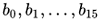, these bytes are shows as this two-dimensional array,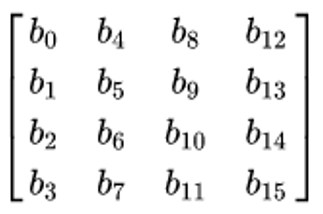The cryptography key size used for an AES cipher specifies the number of transformation rounds that convert the input of a character, called the plaintext or original text, into the final output, called the ciphertext. The number of rounds is as follows as,

• 128-bit keys for 10 rounds.
• 192-bit keys for 12 rounds.
• 256-bit keys for 14 rounds.

## Advanced Encryption Standard (AES) Features

The features of AES are as follows,

• The symmetric key is a symmetric block cipher in cryptography.
• 128-bit of plaintext, and 128/192/256-bit keys for a particular round.
• Rounds are 10,12,14 depend upon key.
• From Triple-DES, AES is Stronger and faster.
• It provides a full specification and design details in this process
• The Software implementable for this AES is in C and Java.

## Advanced Encryption Standard (AES) Working

It is repeated rather than Feistel cipher. It depends on the 'substitution–permutation network' in cryptography. It contains a series of connected operations with each other, some of which involve replacing inputs by specific outputs by substitution and others involve shuffling bits around as permutation in the cryptography.

After that, AES performs all its computations on bytes than bits of character. Hence, AES treats the 128 bits of plaintext or original text block as 16 bytes of blocks. These 16 bytes of plaintext are arranged in four columns and four rows for processing as a matrix of 4X4,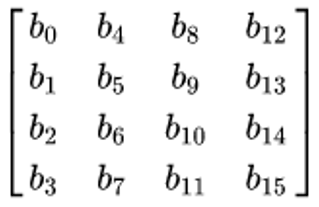Dissimilar to DES, the number of rounds in AES is variant and based on the length of the cryptography key. AES uses 128-bit keys for 10 rounds,192-bit keys for 12 rounds and 256-bit keys for 14 rounds. Each of these rounds uses a different 128-bit round key, which is calculated from the original AES key in the cryptography.

The structure of AES structure is given,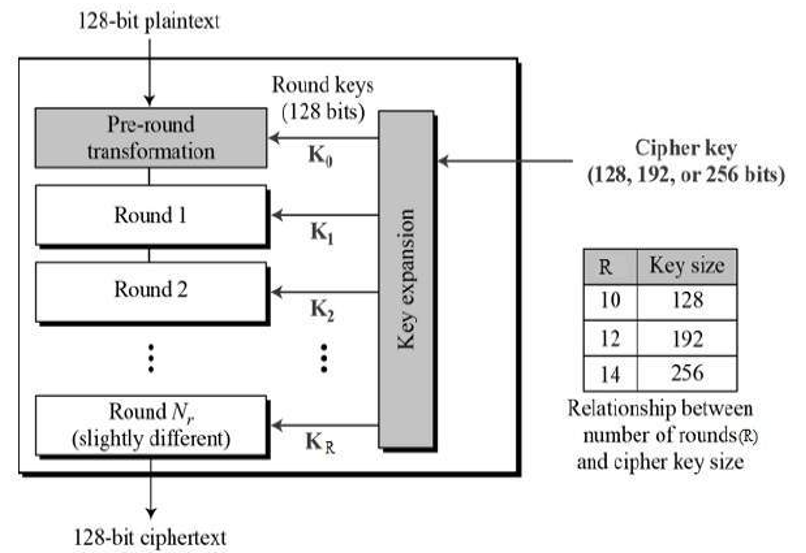Every round consists of several processing steps, including one that depends on the encryption key itself in cryptography. Steps of reverse rounds are applied to convert ciphertext back into the original text or plaintext using the same encryption key in the cryptography.

## AES Encryption Process

In this, we restrict to the description of a typical round of AES encryption. Each round comprises of four sub-processes in the blocks. The first round process is depicted below,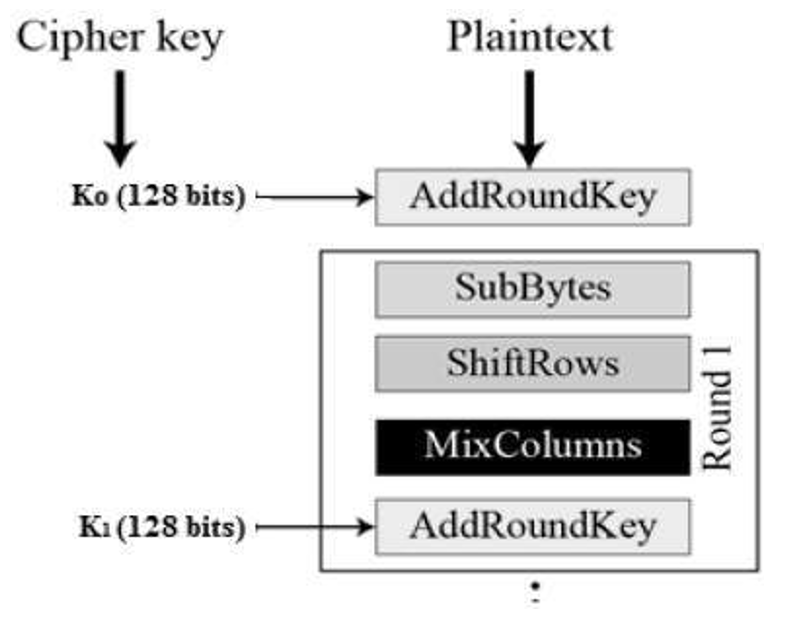### Byte Substitution (SubBytes)

The 16 input bytes of plaintext are substituted by checking up a fixed table (S-box) given in design or the book. The result is in a matrix of four rows and four columns in the four bits used as rows and ending four bits use as a column as by this search in the table and replace the previous one.

### Shiftrows

After the byte substitution, each of the four rows of the matrix is shifted to the left by particular bits. The shift is changing as follows,

• The first row is not shifted to write as it is.
• The second row is shifted by one-byte position to the left side.
• The third row is shifted by two positions to the left side.
• The fourth row is shifted by three positions to the left side.
• The result is a new matrix consisting of the same 16 bytes but shifted concerning each other in this process.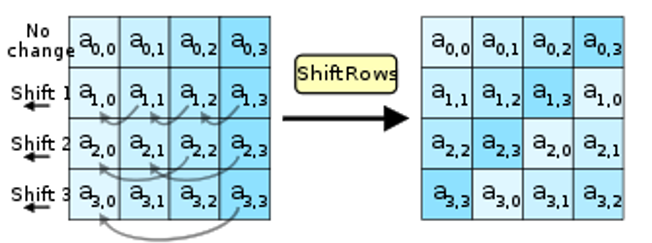### MixColumns

After the previous two steps now, each column of four bytes is now changed using a special mathematical function. These function takes as input the four bytes of one column as the previous answer and outputs four completely new bytes, which replace the original column by this. The result is another new matrix consisting of 16 new bytes that use this mix column. It should be noted that this step is not performed in the last round in the cryptography.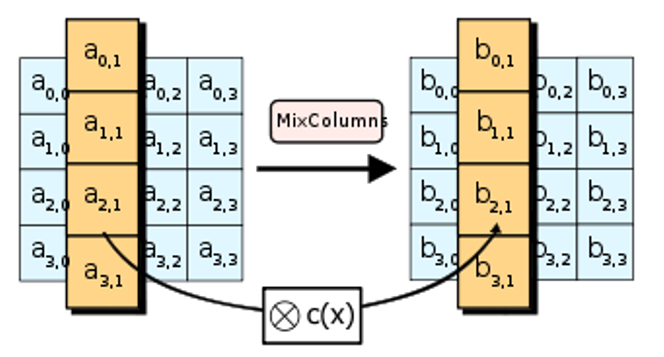The 16 bytes of the matrix are now considered as 128 bits of plaintext and are XORed to the 128 bits of the round cryptography key. If this is the last round then the last output is the ciphertext in the cryptography. Whereas, the resulting 128 bits are interpreted as 16 bytes and we begin another similar round in this.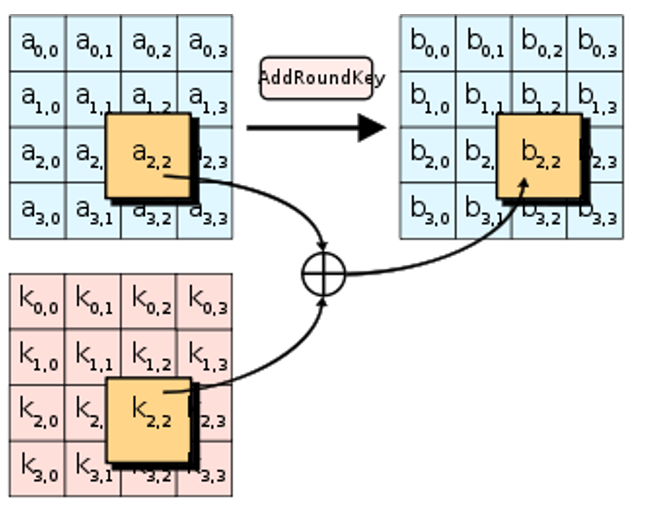## AES Decryption Process

The steps of decryption of an AES ciphertext is the same as to the encryption process in the reverse order in the cryptography. Each of the rounds consists of the four processes conducted in the reverse order in this process,

• Mix columns
• Shift rows
• Byte substitution

This is a process in each round are worked in decryption manner, not similar for a Feistel Structure, the algorithms need to be separately executed, although they are very closely related to each other in the cryptography in the cryptoanalysis.

## AES Analysis

Nowadays cryptography, AES is widely adopted and supported in both hardware and software as the process. Until now a date, no practical cryptanalytic attacks against AES have been discovered in the process. Similarly, AES has built-in flexibility of cryptography key length, which allows a power of "future-proofing" against progress in the ability to perform exhaustive key searches in the cryptography.

Wherever, just as for DES, the AES security is assured only if it is correctly implemented and good key management is employed in the cryptography.

## AES Security

The design and strength of all cryptography key lengths of the AES algorithm i.e., 128, 192 and 256 are sufficient to protect work up to the Secret level. Top Secret information will require the use of either the 192 or 256 cryptography key lengths. This is an execution of AES in products intended to protect from national security systems and work with must be reviewed and certified by NSA before their acquisition and use in the cryptography.

• Known attacks: Mainly cryptographers, a cryptographic "break" is anything faster and stronger than a brute force attack – performing one trial decryption for each possible cryptography key in sequence. This is a keyspace increase by a factor of 2 for each additional bit of cryptography key length, and if every possible value of the key is equiprobable, this translates into a doubling of the average always brute-force key search time in the cryptography.
• Side-channel attacks: Side-Channel attack do not attack the cipher as a black box, and thus are not related to cipher security as cryptography defined in the classical context, but are important in practice in this. This attack implementation of the cipher on hardware or software systems that inadvertently leak data in the cryptography. They are several such known attacks on various implementations of AES in cryptography.

Image references: